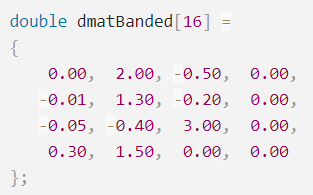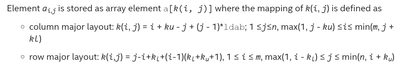Intel® oneAPI Math Kernel Library
Ask questions and share information with other developers who use Intel® Math Kernel Library.
6834 Discussions

## Cannot get LAPACKE_dgbtrf to return a meaningful result for row_major format.Beginner
868 Views

I've tried many variations, but I can't seem to figure out how to format a simple banded matrix in row_major format.  The following code uses a complete 4x4 matrix and finds the LU decomposition, and then solves a simple linear equation.

I'd like to do the same with the banded matrix interface dgbtrf, but I only get nonsense as output.  The documentation for row_major matrices is less complete than for column_major and also contains obvious errors.

So can somebody tell me what the matrix dmatBanded should look like, and what the right parameters are for LAPACKE_dgbtrf?

I have included test code below and its output.  First I use dgetrf on matrix dmat.  Then I try to use dgbtrf on matrix dmatBanded.

Thanks!

PS - Running C++ 2018.2.185 on Windows 7.  Visual Studio 2015.

``````void LinearAlgebra::Test01()
{
using std::cout;
using std::endl;

double dmat =
{
2.00, -0.50,  0.00,  0.00,
-0.01,  1.30, -0.20,  0.00,
0.00, -0.05, -0.40,  3.00,
0.00,  0.00,  0.30,  1.50
};
lapack_int pivots;
PrintMatrix(dmat, 4, 4, "dmat");
lapack_int info = LAPACKE_dgetrf(LAPACK_ROW_MAJOR, 4, 4, dmat, 4, pivots);
PrintMatrix(dmat, 4, 4, "dmat LU");
cout << "info=" << info << " pivots=" << pivots << "," << pivots << "," << pivots << "," << pivots << endl;
double bvec = { 1.0, 2.0, 3.0, 4.0 };
info = LAPACKE_dgetrs(LAPACK_ROW_MAJOR, 'N', 4, 1, dmat, 4, pivots, bvec, 1);
cout << "info=" << info << endl;
PrintMatrix(bvec, 1, 4, "solution");
cout << "-----------------\n\n";

double dmatBanded =
{
0.00,  2.00, -0.50,  0.00,
-0.01,  1.30, -0.20,  0.00,
-0.05, -0.40,  3.00,  0.00,
0.30,  1.50,  0.00,  0.00
};
PrintMatrix(dmatBanded, 4, 4, "dmatBanded");
int rows = 4;
int cols = 4;
int kl = 1;
int ku = 1;
int ldab = 4;
info = LAPACKE_dgbtrf(LAPACK_ROW_MAJOR, rows, cols, kl, ku, dmatBanded, ldab, pivots);
PrintMatrix(dmatBanded, 4, 4, "dmatBanded LU result");
cout << "info=" << info << " pivots=" << pivots << "," << pivots << "," << pivots << "," << pivots << endl;
double bvec2 = { 1.0, 2.0, 3.0, 4.0 };
info = LAPACKE_dgbtrs(LAPACK_ROW_MAJOR, 'N', rows, kl, ku, 1, dmatBanded, ldab, pivots, bvec2, 1);
cout << "info=" << info << endl;
PrintMatrix(bvec2, 1, 4, "solution");
cout << "-----------------\n\n";
}
``````

Here is the output:

``````dmat
2.000000  -0.500000   0.000000   0.000000
-0.010000   1.300000  -0.200000   0.000000
0.000000  -0.050000  -0.400000   3.000000
0.000000   0.000000   0.300000   1.500000

dmat LU
2.000000  -0.500000   0.000000   0.000000
-0.005000   1.297500  -0.200000   0.000000
0.000000  -0.038536  -0.407707   3.000000
0.000000   0.000000  -0.735822   3.707467

info=0 pivots=1,2,3,4
info=0
solution
1.074570   2.298279   4.885086   1.689649

-----------------

dmatBanded
0.000000   2.000000  -0.500000   0.000000
-0.010000   1.300000  -0.200000   0.000000
-0.050000  -0.400000   3.000000   0.000000
0.300000   1.500000   0.000000   0.000000

dmatBanded LU result
0.000000   2.000000  -0.200000   0.000000
-0.010000  -0.400000   3.000000   0.000000
0.300000   1.500000  -2.500000   0.000000
-0.166667   0.822222  -0.000000   0.000000

info=4 pivots=2,3,3,4
info=0
solution
-nan(ind)  -nan(ind)  -nan(ind)        inf

-----------------``````

Oh, here's PrintMatrix if you like.  It is not interesting.

``````void LinearAlgebra::PrintMatrix(double *mat, int n, int m, char *title)
{
using std::cout;
using std::endl;

cout << title << endl;
char buf;
for (int i = 0; i < n; i++)
{
for (int j = 0; j < m; j++)
{
sprintf_s(buf, 128, " %10.6f", mat[i*m+j]);
cout << buf;
}
cout << endl;
}
cout << endl;
}``````

Labels (1)
• ### Code Samples

3 RepliesModerator
845 Views

Hi,

>>The documentation for row_major matrices is less complete than for column_major and also contains obvious errors.

Can you please provide us links where the documentation contains errors and what do you think should be added to the row_major matrices?

>>So can somebody tell me what the matrix dmatBanded should look like, and what the right parameters are for LAPACKE_dgbtrf?

Along with these details can you please provide the MKL Version used?

Please let us know if you have any queries.

Regards

Rajesh.Beginner
820 Views

Here is the first documentation bug that comes to mind.  It's from the "Link for band matrix" that you provided.Of course, the row major layout calculation is silly.  It should not use (kl+ku+1), it should use ldab.  In the case of dgbtrf, ldab must be greater than or equal to (2*kl+ku+1), so that is troublesome.
I might also mention that both the column and row major discussions that precede this always assume i and j are 1-based, as in fortran.  But the indices calculated with these two formulae for the location in a[k(i,j)] are zero-based.  This is confusing.

So I have referred to the documentation you reference dozens of times, and it is what led me to the code that I wrote.  It's all about this:Is this the correct order for the entries in a row_major 4x4 banded matrix?  As I read and interpret the documentation, it is correct.

I am using the MKL that came with C++ 2018.2.185, so it seems to have the same version number.  I am static linking to the MKL, and using 32 bit integers.  You can see in my code that the library works, until I get to the banded matrix call.

Thanks,

ChuckBeginner
806 Views

OK, I am going to have to solve this myself.
Summary: The Intel MKL documentation relating to banded matrix storage in row major format is badly misleading.  Which is to say it is very very wrong.

For row major storage, like I use in C++, they say the banded version of a stored matrix should look like this:Some things to note:
1) The main diagonal of the original matrix a[1,1], a[2,2], etc, appears as a column in the banded version.
2) The first sub-diagonal, a[2,1], a[3,2], etc, is a column left of the main diagonal. Also it is displaced downward by one row compared to the main diagonal.
3) The first super-diagonal, a[1,2], a[2,3], etc, appears in a column to the right of the main diagonal and is even with the top of the main diagonal.
This is pretty clear from the picture, right?
Then for dgbtrf I know that I need to reserve another super diagonal for the output.  So that puts another column on the right.

Let's apply this knowledge.
This is my full matrix, as posted yesterday:So following the three points above, I construct my version of the banded matrix:As in the documentation: "Diagonals of A become columns of AB".
Consider also the formula given a little below in the same document:Look at the row major layout formula and consider where diagonal elements go.  Note that i=j, so that the next sequential diagonal element should be located according to kl+(i-1)(kl+ku+1).  In particular, it is located (kl+ku+1) away in the flattened array. (And that term is silly in itself. ldab is more likely.)

I have followed the instructions carefully, and arrived where I did.  By searching the internet, I have found that every couple of years a confused person posts this question, and they have tried an array definition just like mine, and they have failed.

So: All of the above documentation is completely wrong.

Here is the matrix that actually works for LPACK_ROW_MAJOR format:Note that the main diagonal is a row, not a column.

Note that sequential diagonal elements are stored sequentially in memory, not separated by ldab.

Note that the sub-diagonal is aligned with the main diagonal, not offset.  And vice versa for the super-diagonal.

Here is a code sample in which it works:

``````void LinearAlgebra::Test01()
{
using std::cout;
using std::endl;

double dmat =
{
2.00, -0.50,  0.00,  0.00,
-0.01,  1.30, -0.20,  0.00,
0.00, -0.05, -0.40,  3.00,
0.00,  0.00,  0.30,  1.50
};
lapack_int pivots;
PrintMatrix(dmat, 4, 4, "dmat");
lapack_int info = LAPACKE_dgetrf(LAPACK_ROW_MAJOR, 4, 4, dmat, 4, pivots);
PrintMatrix(dmat, 4, 4, "dmat LU");
cout << "info=" << info << " pivots=" << pivots << "," << pivots << "," << pivots << "," << pivots << endl;
double bvec = { 1.0, 2.0, 3.0, 4.0 };
info = LAPACKE_dgetrs(LAPACK_ROW_MAJOR, 'N', 4, 1, dmat, 4, pivots, bvec, 1);
cout << "info=" << info << endl;
PrintMatrix(bvec, 1, 4, "solution");
cout << "-----------------\n\n";

double dmatBanded =
{
0.00,  0.00,  0.00, 0.00,
0.00, -0.50, -0.20, 3.00,
2.00,  1.30, -0.40, 1.50,
-0.01, -0.05,  0.30, 0.00
};
PrintMatrix(dmatBanded, 4, 4, "dmatBanded");
int rows = 4;
int cols = 4;
int kl = 1;
int ku = 1;
int ldab = 4;
info = LAPACKE_dgbtrf(LAPACK_ROW_MAJOR, rows, cols, kl, ku, dmatBanded, ldab, pivots);
PrintMatrix(dmatBanded, 4, 4, "dmatBanded LU result");
cout << "info=" << info << " pivots=" << pivots << "," << pivots << "," << pivots << "," << pivots << endl;
double bvec2 = { 1.0, 2.0, 3.0, 4.0 };
info = LAPACKE_dgbtrs(LAPACK_ROW_MAJOR, 'N', rows, kl, ku, 1, dmatBanded, ldab, pivots, bvec2, 1);
cout << "info=" << info << endl;
PrintMatrix(bvec2, 1, 4, "solution");
cout << "-----------------\n\n";

double dmatBandedCol =
{
0.00,  0.00,  2.00, -0.01,
0.00, -0.50,  1.30, -0.05,
0.00, -0.20, -0.40,  0.30,
0.00,  3.00,  1.50,  0.00
};
PrintMatrix(dmatBandedCol, 4, 4, "dmatBanded column major");
rows = 4;
cols = 4;
kl = 1;
ku = 1;
ldab = 4;
info = LAPACKE_dgbtrf(LAPACK_COL_MAJOR, rows, cols, kl, ku, dmatBandedCol, ldab, pivots);
PrintMatrix(dmatBandedCol, 4, 4, "dmatBandedCol LU result");
cout << "info=" << info << " pivots=" << pivots << "," << pivots << "," << pivots << "," << pivots << endl;
double bvec3 = { 1.0, 2.0, 3.0, 4.0 };
info = LAPACKE_dgbtrs(LAPACK_COL_MAJOR, 'N', rows, kl, ku, 1, dmatBandedCol, ldab, pivots, bvec3, 4);
cout << "info=" << info << endl;
PrintMatrix(bvec3, 1, 4, "solution");
cout << "-----------------\n\n";
}
``````

Here is the output of that code:

``````dmat
2.000000  -0.500000   0.000000   0.000000
-0.010000   1.300000  -0.200000   0.000000
0.000000  -0.050000  -0.400000   3.000000
0.000000   0.000000   0.300000   1.500000

dmat LU
2.000000  -0.500000   0.000000   0.000000
-0.005000   1.297500  -0.200000   0.000000
0.000000  -0.038536  -0.407707   3.000000
0.000000   0.000000  -0.735822   3.707467

info=0 pivots=1,2,3,4
info=0
solution
1.074570   2.298279   4.885086   1.689649

-----------------

dmatBanded
0.000000   0.000000   0.000000   0.000000
0.000000  -0.500000  -0.200000   3.000000
2.000000   1.300000  -0.400000   1.500000
-0.010000  -0.050000   0.300000   0.000000

dmatBanded LU result
0.000000   0.000000   0.000000   0.000000
0.000000  -0.500000  -0.200000   3.000000
2.000000   1.297500  -0.407707   3.707467
-0.005000  -0.038536  -0.735822   0.000000

info=0 pivots=1,2,3,4
info=0
solution
1.074570   2.298279   4.885086   1.689649

-----------------

dmatBanded column major
0.000000   0.000000   2.000000  -0.010000
0.000000  -0.500000   1.300000  -0.050000
0.000000  -0.200000  -0.400000   0.300000
0.000000   3.000000   1.500000   0.000000

dmatBandedCol LU result
0.000000   0.000000   2.000000  -0.005000
0.000000  -0.500000   1.297500  -0.038536
0.000000  -0.200000  -0.407707  -0.735822
0.000000   3.000000   3.707467   0.000000

info=0 pivots=1,2,3,4
info=0
solution
1.074570   2.298279   4.885086   1.689649

-----------------``````

I suspect that there are no code samples on the net for using LAPACK_ROW_MAJOR banded matrices from C or C++ because people struggle with it for a while and then give up.  I hope this code sample gets found by the next person.

Regards,

Chuck